## Section 1.9: Transformation of Functions

### Learning Outcomes

• Graph functions using a single transformation.
• Graph functions using a combination of transformations.
• Describe transformations based on a function formula.
• Give the formula of a function based on its transformations.

We all know that a flat mirror enables us to see an accurate image of ourselves and whatever is behind us. When we tilt the mirror, the images we see may shift horizontally or vertically. But what happens when we bend a flexible mirror? Like a carnival funhouse mirror, it presents us with a distorted image of ourselves, stretched or compressed horizontally or vertically. In a similar way, we can distort or transform mathematical functions to better adapt them to describing objects or processes in the real world. In this section, we will take a look at several kinds of transformations.

## Graphing Functions Using Vertical and Horizontal Shifts

Often when given a problem, we try to model the scenario using mathematics in the form of words, tables, graphs, and equations. One method we can employ is to adapt the basic graphs of the toolkit functions to build new models for a given scenario. There are systematic ways to alter functions to construct appropriate models for the problems we are trying to solve.

## Identifying Vertical Shifts

One simple kind of transformation involves shifting the entire graph of a function up, down, right, or left. The simplest shift is a vertical shift, moving the graph up or down, because this transformation involves adding a positive or negative constant to the function. In other words, we add the same constant to the output value of the function regardless of the input. For a function $g\left(x\right)=f\left(x\right)+k$, the function $f\left(x\right)$ is shifted vertically $k$ units.Figure 2. Vertical shift by $k=1$ of the cube root function $f\left(x\right)=\sqrt{x}$.

To help you visualize the concept of a vertical shift, consider that $y=f\left(x\right)$. Therefore, $f\left(x\right)+k$ is equivalent to $y+k$. Every unit of $y$ is replaced by $y+k$, so the $y\text{-}$ value increases or decreases depending on the value of $k$. The result is a shift upward or downward.

### A General Note: Vertical Shift

Given a function $f\left(x\right)$, a new function $g\left(x\right)=f\left(x\right)+k$, where $k$ is a constant, is a vertical shift of the function $f\left(x\right)$. All the output values change by $k$ units. If $k$ is positive, the graph will shift up. If $k$ is negative, the graph will shift down.

### Example 1: Adding a Constant to a Function

To regulate temperature in a green building, airflow vents near the roof open and close throughout the day. Figure 2 shows the area of open vents $V$ (in square feet) throughout the day in hours after midnight, $t$. During the summer, the facilities manager decides to try to better regulate temperature by increasing the amount of open vents by 20 square feet throughout the day and night. Sketch a graph of this new function.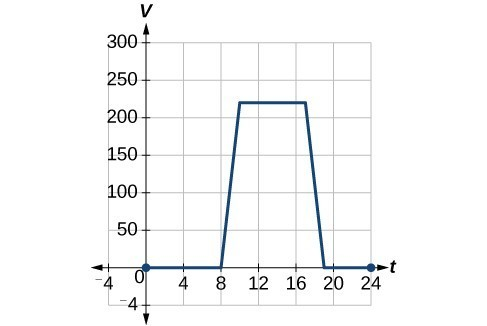### How To: Given a tabular function, create a new row to represent a vertical shift.

1. Identify the output row or column.
2. Determine the magnitude of the shift.
3. Add the shift to the value in each output cell. Add a positive value for up or a negative value for down.

### Example 2: Shifting a Tabular Function Vertically

A function $f\left(x\right)$ is given below. Create a table for the function $g\left(x\right)=f\left(x\right)-3$.

 $x$ 2 4 6 8 $f\left(x\right)$ 1 3 7 11

## Identifying Horizontal Shifts

We just saw that the vertical shift is a change to the output, or outside, of the function. We will now look at how changes to input, on the inside of the function, change its graph and meaning. A shift to the input results in a movement of the graph of the function left or right in what is known as a horizontal shift.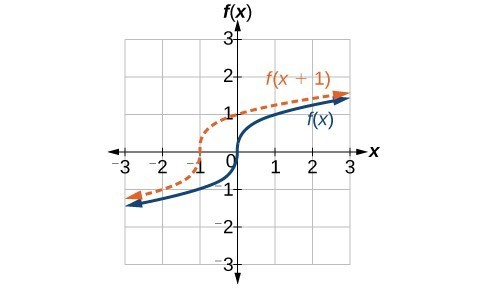Figure 5. Horizontal shift of the function $f\left(x\right)=\sqrt{x}$. Note that $h=+1$ shifts the graph to the left, that is, towards negative values of $x$.

For example, if $f\left(x\right)={x}^{2}$, then $g\left(x\right)={\left(x - 2\right)}^{2}$ is a new function. Each input is reduced by 2 prior to squaring the function. The result is that the graph is shifted 2 units to the right, because we would need to increase the prior input by 2 units to yield the same output value as given in $f$.

### A General Note: Horizontal Shift

Given a function $f$, a new function $g\left(x\right)=f\left(x-h\right)$, where $h$ is a constant, is a horizontal shift of the function $f$. If $h$ is positive, the graph will shift right. If $h$ is negative, the graph will shift left.

### Example 3: Adding a Constant to an Input

Returning to our building airflow example from Example 2, suppose that in autumn the facilities manager decides that the original venting plan starts too late, and wants to begin the entire venting program 2 hours earlier. Sketch a graph of the new function.

### How To: Given a tabular function, create a new row to represent a horizontal shift.

1. Identify the input row or column.
2. Determine the magnitude of the shift.
3. Add the shift to the value in each input cell.

### Example 4: Shifting a Tabular Function Horizontally

A function $f\left(x\right)$ is given below. Create a table for the function $g\left(x\right)=f\left(x - 3\right)$.

 $x$ 2 4 6 8 $f\left(x\right)$ 1 3 7 11

### Example 5: Identifying a Horizontal Shift of a Toolkit Function

This graph represents a transformation of the toolkit function $f\left(x\right)={x}^{2}$. Relate this new function $g\left(x\right)$ to $f\left(x\right)$, and then find a formula for $g\left(x\right)$.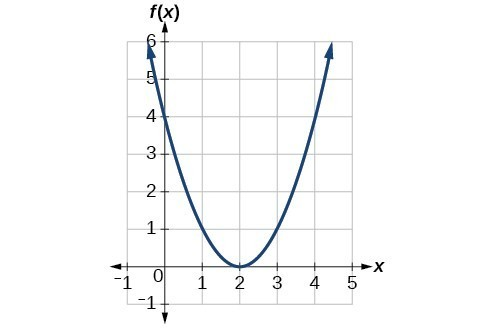Figure 8

### Example 6: Interpreting Horizontal versus Vertical Shifts

The function $G\left(m\right)$ gives the number of gallons of gas required to drive $m$ miles. Interpret $G\left(m\right)+10$ and $G\left(m+10\right)$.

### Try It

Given the function $f\left(x\right)=\sqrt{x}$, graph the original function $f\left(x\right)$ and the transformation $g\left(x\right)=f\left(x+2\right)$ on the same axes. Is this a horizontal or a vertical shift? Which way is the graph shifted and by how many units?

## Graphing Functions Using Reflections about the Axes

Another transformation that can be applied to a function is a reflection over the x– or y-axis. A vertical reflection reflects a graph vertically across the x-axis, while a horizontal reflection reflects a graph horizontally across the y-axis. The reflections are shown in Figure 9.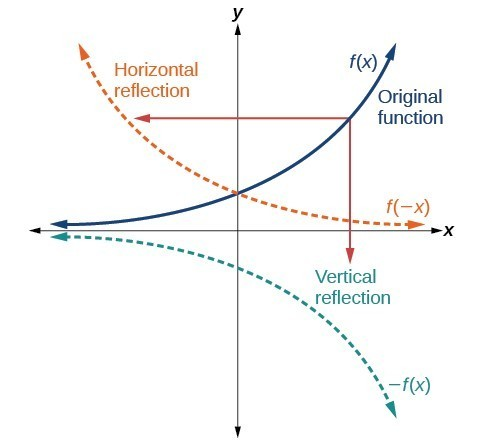Figure 9. Vertical and horizontal reflections of a function.

Notice that the vertical reflection produces a new graph that is a mirror image of the base or original graph about the x-axis. The horizontal reflection produces a new graph that is a mirror image of the base or original graph about the y-axis.

### A General Note: Reflections

Given a function $f\left(x\right)$, the function $g\left(x\right)=-f\left(x\right)$ is a vertical reflection of the function $f\left(x\right)$, sometimes called a reflection about (or over, or through) the x-axis.

Given a function $f\left(x\right)$, the function $g\left(x\right)=f\left(-x\right)$ is a horizontal reflection of the function $f\left(x\right)$, sometimes called a reflection about the y-axis.

### How To: Given a function, reflect the graph both vertically and horizontally.

1. Multiply all outputs by –1 for a vertical reflection. This is the same as multiplying the entire function by -1. The new graph is a reflection of the original graph about the x-axis.
2. Multiply all inputs by –1 for a horizontal reflection. This is the same as multiplying the input variable by -1. The new graph is a reflection of the original graph about the y-axis.

### Example 7: Reflecting a Graph Horizontally and Vertically

Reflect the graph of $s\left(t\right)=\sqrt{t}$ (a) vertically and (b) horizontally.

### Try It

Reflect the graph of $f\left(x\right)=|x - 1|$ (a) vertically and (b) horizontally.

### Example 8: Reflecting a Tabular Function Horizontally and Vertically

A function $f\left(x\right)$ is given. Create a table for the functions below.

1. $g\left(x\right)=-f\left(x\right)$
2. $h\left(x\right)=f\left(-x\right)$
 $x$ 2 4 6 8 $f\left(x\right)$ 1 3 7 11

### Try It

 $x$ −2 0 2 4 $f\left(x\right)$ 5 10 15 20

Using the function $f\left(x\right)$ given in the table above, create a table for the functions below.

a. $g\left(x\right)=-f\left(x\right)$

b. $h\left(x\right)=f\left(-x\right)$

## Graphing Functions Using Stretches and Compressions

Adding a constant to the inputs or outputs of a function changed the position of a graph with respect to the axes, but it did not affect the shape of a graph. We now explore the effects of multiplying the inputs or outputs by some quantity.

We can transform the inside (input values) of a function or we can transform the outside (output values) of a function. Each change has a specific effect that can be seen graphically.

## Vertical Stretches and Compressions

When we multiply a function by a positive constant, we get a function whose graph is stretched or compressed vertically in relation to the graph of the original function. If the constant is greater than 1, we get a vertical stretch; if the constant is between 0 and 1, we get a vertical compression. The graph below shows a function multiplied by constant factors 2 and 0.5 and the resulting vertical stretch and compression.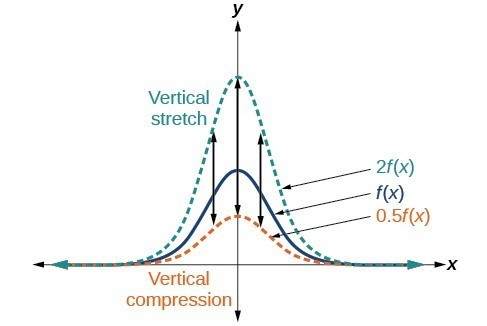Figure 12. Vertical stretch and compression

### A General Note: Vertical Stretches and Compressions

Given a function $f\left(x\right)$, a new function $g\left(x\right)=af\left(x\right)$, where $a$ is a constant, is a vertical stretch or vertical compression of the function $f\left(x\right)$.

• If $a>1$, then the graph will be stretched.
• If 0 < a < 1, then the graph will be compressed.
• If $a<0$, then there will be combination of a vertical stretch or compression with a vertical reflection.

### How To: Given a function, graph its vertical stretch.

1. Identify the value of $a$.
2. Multiply all range values by $a$.
3. If $a>1$, the graph is stretched by a factor of $a$.

If ${ 0 }<{ a }<{ 1 }$, the graph is compressed by a factor of $a$.

If $a<0$, the graph is either stretched or compressed and also reflected about the x-axis.

### Example 9: Graphing a Vertical Stretch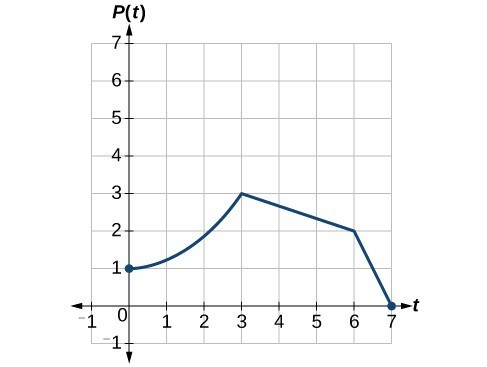Figure 13

A function $P\left(t\right)$ models the population of fruit flies.

A scientist is comparing this population to another population, $Q$, whose growth follows the same pattern, but is twice as large. Sketch a graph of this population.

### How To: Given a tabular function and assuming that the transformation is a vertical stretch or compression, create a table for a vertical compression.

1. Determine the value of $a$.
2. Multiply all of the output values by $a$.

### Example 10: Finding a Vertical Compression of a Tabular Function

A function $f$ is given in the table below. Create a table for the function $g\left(x\right)=\frac{1}{2}f\left(x\right)$.

 $x$ 2 4 6 8 $f\left(x\right)$ 1 3 7 11

### Try It

A function $f$ is given below. Create a table for the function $g\left(x\right)=\frac{3}{4}f\left(x\right)$.

 $x$ 2 4 6 8 $f\left(x\right)$ 12 16 20 0

### Example 11: Recognizing a Vertical Stretch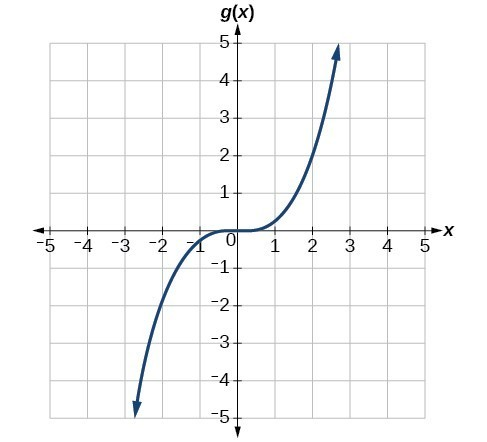Figure 15

The graph is a transformation of the toolkit function $f\left(x\right)={x}^{3}$. Relate this new function $g\left(x\right)$ to $f\left(x\right)$, and then find a formula for $g\left(x\right)$.

### Try It

Write the formula for the function that we get when we stretch the identity toolkit function by a factor of 3, and then shift it down by 2 units.

## Horizontal Stretches and Compressions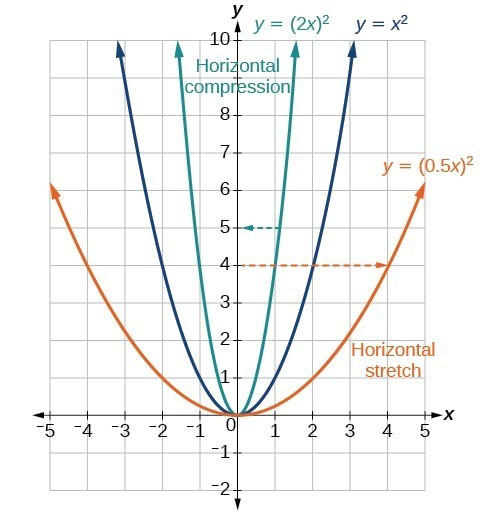Figure 16

Now we consider changes to the inside of a function. When we multiply a function’s input by a positive constant, we get a function whose graph is stretched or compressed horizontally in relation to the graph of the original function. If the constant is between 0 and 1, we get a horizontal stretch; if the constant is greater than 1, we get a horizontal compression of the function.

Given a function $y=f\left(x\right)$, the form $y=f\left(bx\right)$ results in a horizontal stretch or compression. Consider the function $y={x}^{2}$. The graph of $y={\left(0.5x\right)}^{2}$ is a horizontal stretch of the graph of the function $y={x}^{2}$ by a factor of 2. The graph of $y={\left(2x\right)}^{2}$ is a horizontal compression of the graph of the function $y={x}^{2}$ by a factor of 2.

### A General Note: Horizontal Stretches and Compressions

Given a function $f\left(x\right)$, a new function $g\left(x\right)=f\left(bx\right)$, where $b$ is a constant, is a horizontal stretch or horizontal compression of the function $f\left(x\right)$.

• If $b>1$, then the graph will be compressed by $\frac{1}{b}$.
• If $0<b<1$, then the graph will be stretched by $\frac{1}{b}$.
• If $b<0$, then there will be combination of a horizontal stretch or compression with a horizontal reflection.

### How To: Given a description of a function, sketch a horizontal compression or stretch.

1. Write a formula to represent the function.
2. Set $g\left(x\right)=f\left(bx\right)$ where $b>1$ for a compression or $0<b<1$
for a stretch.

### Example 12: Graphing a Horizontal Compression

Suppose a scientist is comparing a population of fruit flies to a population that progresses through its lifespan twice as fast as the original population. In other words, this new population, $R$, will progress in 1 hour the same amount as the original population does in 2 hours, and in 2 hours, it will progress as much as the original population does in 4 hours. Sketch a graph of this population.

### Example 13: Finding a Horizontal Stretch for a Tabular Function

A function $f\left(x\right)$ is given below. Create a table for the function $g\left(x\right)=f\left(\frac{1}{2}x\right)$.

 $x$ 2 4 6 8 $f\left(x\right)$ 1 3 7 11

### Example 14: Recognizing a Horizontal Compression on a Graph

Relate the function $g\left(x\right)$ to $f\left(x\right)$ in Figure 19.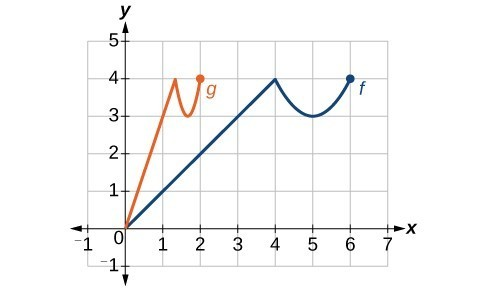Figure 19

### Try It

Write a formula for the toolkit square root function horizontally stretched by a factor of 3.

## Combining Vertical and Horizontal Shifts

Now that we have two transformations, we can combine them together. Vertical shifts are outside changes that affect the output ( $y\text{-}$ ) axis values and shift the function up or down. Horizontal shifts are inside changes that affect the input ( $x\text{-}$ ) axis values and shift the function left or right. Combining the two types of shifts will cause the graph of a function to shift up or down and right or left.

### How To: Given a function and both a vertical and a horizontal shift, sketch the graph.

1. Identify the vertical and horizontal shifts from the formula.
2. The vertical shift results from a constant added to the output. Move the graph up for a positive constant and down for a negative constant.
3. The horizontal shift results from a constant added to the input. Move the graph left for a positive constant and right for a negative constant.
4. Apply the shifts to the graph in either order.

### Example 15: Graphing Combined Vertical and Horizontal Shifts

Given $f\left(x\right)=|x|$, sketch a graph of $h\left(x\right)=f\left(x+1\right)-3$.

### Try It

Given $f\left(x\right)=|x|$, sketch a graph of $h\left(x\right)=f\left(x - 2\right)+4$.

### Example 16: Identifying Combined Vertical and Horizontal Shifts

Write a formula for the graph shown in Figure 22, which is a transformation of the toolkit square root function.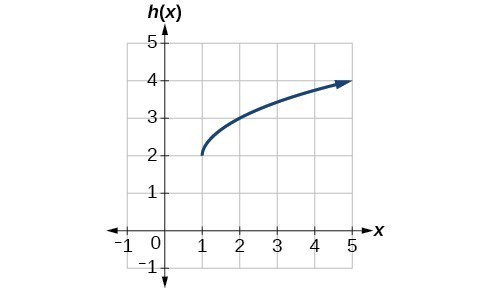Figure 22

### Try It

Write a formula for a transformation of the toolkit reciprocal function $f\left(x\right)=\frac{1}{x}$ that shifts the function’s graph one unit to the right and one unit up.

### Example 17: Applying a Learning Model Equation

A common model for learning has an equation similar to $k\left(t\right)=-{2}^{-t}+1$, where $k$ is the percentage of mastery that can be achieved after $t$ practice sessions. This is a transformation of the function $f\left(t\right)={2}^{t}$ shown in Figure 23. Sketch a graph of $k\left(t\right)$.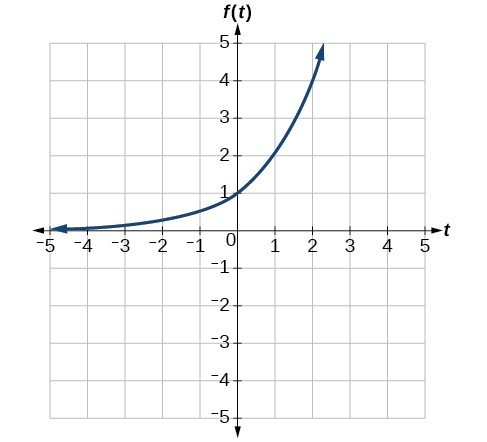Figure 23

### Try It

Given the toolkit function $f\left(x\right)={x}^{2}$, graph $g\left(x\right)=-f\left(x\right)$ and $h\left(x\right)=f\left(-x\right)$. Take note of any surprising behavior for these functions.

## Performing a Sequence of Transformations

When combining transformations, it is very important to consider the order of the transformations. For example, vertically shifting by 3 and then vertically stretching by 2 does not create the same graph as vertically stretching by 2 and then vertically shifting by 3, because when we shift first, both the original function and the shift get stretched, while only the original function gets stretched when we stretch first.

When we see an expression such as $2f\left(x\right)+3$, which transformation should we start with? The answer here follows nicely from the order of operations. Given the output value of $f\left(x\right)$, we first multiply by 2, causing the vertical stretch, and then add 3, causing the vertical shift. In other words, multiplication before addition.

Horizontal transformations are a little trickier to think about. When we write $g\left(x\right)=f\left(2x+3\right)$, for example, we have to think about how the inputs to the function $g$ relate to the inputs to the function $f$. Suppose we know $f\left(7\right)=12$. What input to $g$ would produce that output? In other words, what value of $x$ will allow $g\left(x\right)=f\left(2x+3\right)=12?$ We would need $2x+3=7$. To solve for $x$, we would first subtract 3, resulting in a horizontal shift, and then divide by 2, causing a horizontal compression.

This format ends up being very difficult to work with, because it is usually much easier to horizontally stretch a graph before shifting. We can work around this by factoring inside the function.

$f\left(bx+p\right)=f\left(b\left(x+\frac{p}{b}\right)\right)$

Let’s work through an example.

$f\left(x\right)={\left(2x+4\right)}^{2}$

We can factor out a 2.

$f\left(x\right)={\left(2\left(x+2\right)\right)}^{2}$

Now we can more clearly observe a horizontal shift to the left 2 units and a horizontal compression. Factoring in this way allows us to horizontally stretch first and then shift horizontally.

### A General Note: Combining Transformations

When combining vertical transformations written in the form $af\left(x\right)+k$, first vertically stretch by $a$ and then vertically shift by $k$.

When combining horizontal transformations written in the form $f\left(bx+h\right)$, first horizontally shift by $h$ and then horizontally stretch by $\frac{1}{b}$.

When combining horizontal transformations written in the form $f\left(b\left(x+h\right)\right)$, first horizontally stretch by $\frac{1}{b}$ and then horizontally shift by $h$.

Horizontal and vertical transformations are independent. It does not matter whether horizontal or vertical transformations are performed first.

### Example 18: Finding a Triple Transformation of a Tabular Function

Given the table below for the function $f\left(x\right)$, create a table of values for the function $g\left(x\right)=2f\left(3x\right)+1$.

 $x$ 6 12 18 24 $f\left(x\right)$ 10 14 15 17

### Example 19: Finding a Triple Transformation of a Graph

Use the graph of $f\left(x\right)$ to sketch a graph of $k\left(x\right)=f\left(\frac{1}{2}x+1\right)-3$.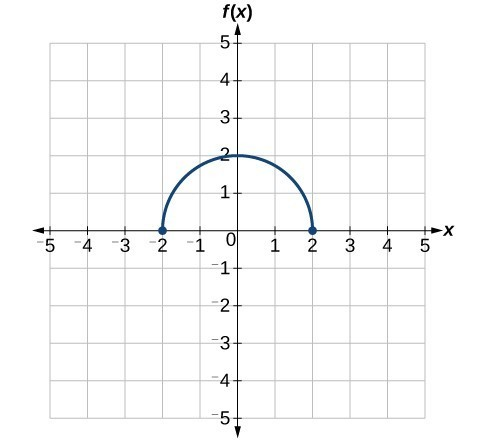Figure 26

## Key Equations

 Vertical shift $g\left(x\right)=f\left(x\right)+k$ (up for $k>0$ ) Horizontal shift $g\left(x\right)=f\left(x-h\right)$ (right for $h>0$ ) Vertical reflection $g\left(x\right)=-f\left(x\right)$ Horizontal reflection $g\left(x\right)=f\left(-x\right)$ Vertical stretch $g\left(x\right)=af\left(x\right)$ ( $a>0$) Vertical compression $g\left(x\right)=af\left(x\right)$ $\left(01$ )

## Key Concepts

• A function can be shifted vertically by adding a constant to the output.
• A function can be shifted horizontally by adding a constant to the input.
• Relating the shift to the context of a problem makes it possible to compare and interpret vertical and horizontal shifts.
• Vertical and horizontal shifts are often combined.
• A vertical reflection reflects a graph about the $x\text{-}$ axis. A graph can be reflected vertically by multiplying the output by –1.
• A horizontal reflection reflects a graph about the $y\text{-}$ axis. A graph can be reflected horizontally by multiplying the input by –1.
• A graph can be reflected both vertically and horizontally. The order in which the reflections are applied does not affect the final graph.
• A function presented in tabular form can also be reflected by multiplying the values in the input and output rows or columns accordingly.
• A function presented as an equation can be reflected by applying transformations one at a time.
• A function can be compressed or stretched vertically by multiplying the output by a constant.
• A function can be compressed or stretched horizontally by multiplying the input by a constant.
• The order in which different transformations are applied does affect the final function. Both vertical and horizontal transformations must be applied in the order given. However, a vertical transformation may be combined with a horizontal transformation in any order.

## Glossary

horizontal compression
a transformation that compresses a function’s graph horizontally, by multiplying the input by a constant $b>1$
horizontal reflection
a transformation that reflects a function’s graph across the y-axis by multiplying the input by $-1$
horizontal shift
a transformation that shifts a function’s graph left or right by adding a positive or negative constant to the input
horizontal stretch
a transformation that stretches a function’s graph horizontally by multiplying the input by a constant $0<b<1$
vertical compression
a function transformation that compresses the function’s graph vertically by multiplying the output by a constant $0<a<1$
vertical reflection
a transformation that reflects a function’s graph across the x-axis by multiplying the output by $-1$
vertical shift
a transformation that shifts a function’s graph up or down by adding a positive or negative constant to the output
vertical stretch
a transformation that stretches a function’s graph vertically by multiplying the output by a constant $a>1$

## Section 1.9 Homework Exercises

1. When examining the formula of a function that is the result of multiple transformations, how can you tell a horizontal shift from a vertical shift?

2. When examining the formula of a function that is the result of multiple transformations, how can you tell a horizontal stretch from a vertical stretch?

3. When examining the formula of a function that is the result of multiple transformations, how can you tell a horizontal compression from a vertical compression?

4. When examining the formula of a function that is the result of multiple transformations, how can you tell a reflection with respect to the x-axis from a reflection with respect to the y-axis?

5. How can you determine whether a function is odd or even from the formula of the function?

6. Write a formula for the function obtained when the graph of $f\left(x\right)=\sqrt{x}$ is shifted up 1 unit and to the left 2 units.

7. Write a formula for the function obtained when the graph of $f\left(x\right)=|x|$
is shifted down 3 units and to the right 1 unit.

8. Write a formula for the function obtained when the graph of $f\left(x\right)=\frac{1}{x}$ is shifted down 4 units and to the right 3 units.

9. Write a formula for the function obtained when the graph of $f\left(x\right)=\frac{1}{{x}^{2}}$ is shifted up 2 units and to the left 4 units.

For the following exercises, describe how the graph of the function is a transformation of the graph of the original function $f$.

10. $y=f\left(x - 49\right)$

11. $y=f\left(x+43\right)$

12. $y=f\left(x+3\right)$

13. $y=f\left(x - 4\right)$

14. $y=f\left(x\right)+5$

15. $y=f\left(x\right)+8$

16. $y=f\left(x\right)-2$

17. $y=f\left(x\right)-7$

18. $y=f\left(x - 2\right)+3$

19. $y=f\left(x+4\right)-1$

For the following exercises, determine the interval(s) on which the function is increasing and decreasing.

20. $f\left(x\right)=4{\left(x+1\right)}^{2}-5$

21. $g\left(x\right)=5{\left(x+3\right)}^{2}-2$

22. $a\left(x\right)=\sqrt{-x+4}$

23. $k\left(x\right)=-3\sqrt{x}-1$

For the following exercises, use the graph of $f\left(x\right)={2}^{x}$ to sketch a graph of each transformation of $f\left(x\right)$.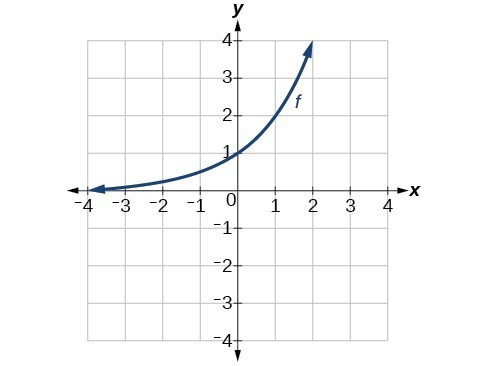24. $g\left(x\right)={2}^{x}+1$

25. $h\left(x\right)={2}^{x}-3$

26. $w\left(x\right)={2}^{x - 1}$

For the following exercises, sketch a graph of the function as a transformation of the graph of one of the toolkit functions.

27. $f\left(t\right)={\left(t+1\right)}^{2}-3$

28. $h\left(x\right)=|x - 1|+4$

29. $k\left(x\right)={\left(x - 2\right)}^{3}-1$

30. $m\left(t\right)=3+\sqrt{t+2}$

31. Tabular representations for the functions $f,g$, and $h$ are given below. Write $g\left(x\right)$ and $h\left(x\right)$ as transformations of $f\left(x\right)$.

 $x$ −2 −1 0 1 2 $f\left(x\right)$ −2 −1 −3 1 2
 $x$ −1 0 1 2 3 $g\left(x\right)$ −2 −1 −3 1 2
 $x$ −2 −1 0 1 2 $h\left(x\right)$ −1 0 −2 2 3

32. Tabular representations for the functions $f,g$, and $h$ are given below. Write $g\left(x\right)$ and $h\left(x\right)$ as transformations of $f\left(x\right)$.

 $x$ −2 −1 0 1 2 $f\left(x\right)$ −1 −3 4 2 1
 $x$ −3 −2 −1 0 1 $g\left(x\right)$ −1 −3 4 2 1
 $x$ −2 −1 0 1 2 $h\left(x\right)$ −2 −4 3 1 0

For the following exercises, write an equation for each graphed function by using transformations of the graphs of one of the toolkit functions.

33.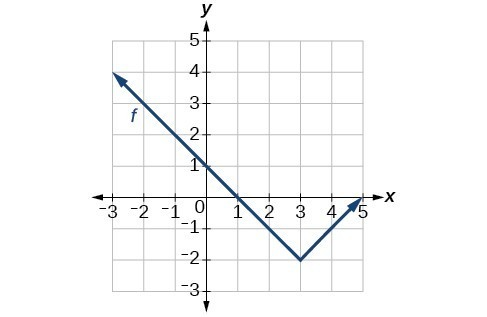34.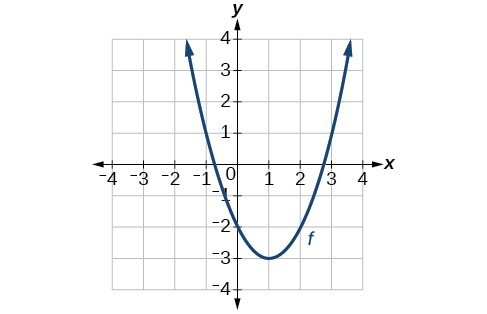35.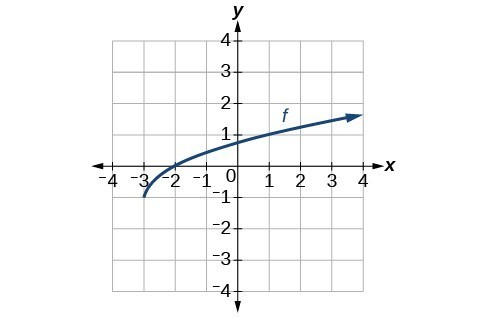36.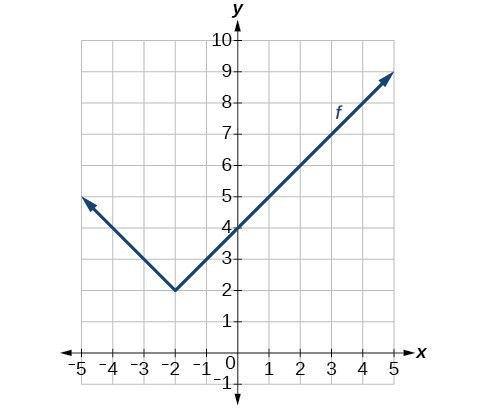37.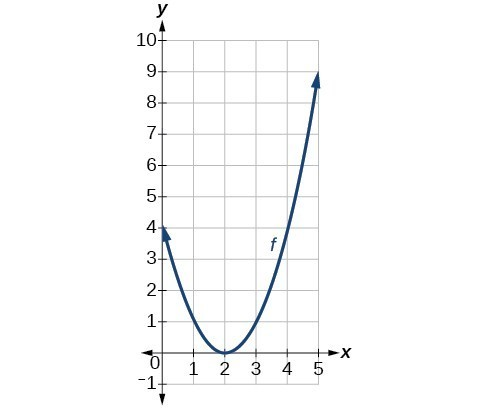38.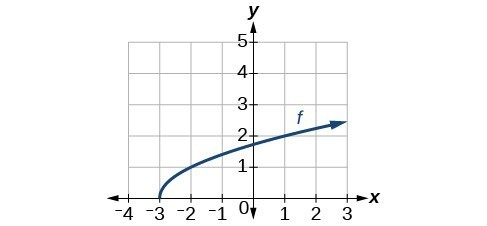39.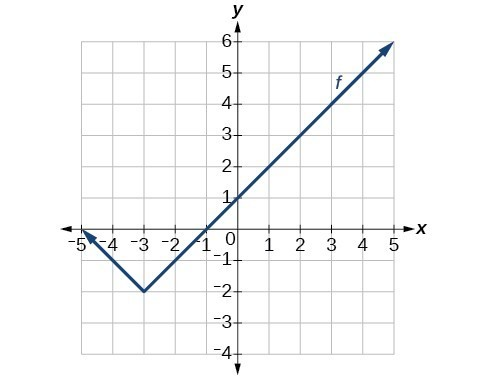40.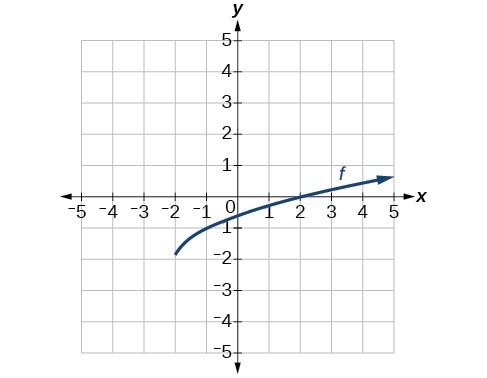For the following exercises, use the graphs of transformations of the square root function to find a formula for each of the functions.

41.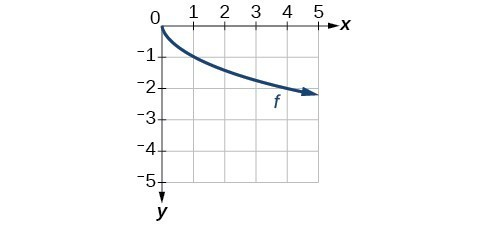42.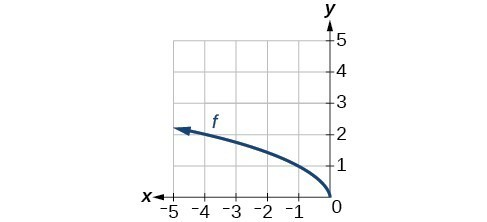For the following exercises, use the graphs of the transformed toolkit functions to write a formula for each of the resulting functions.

43.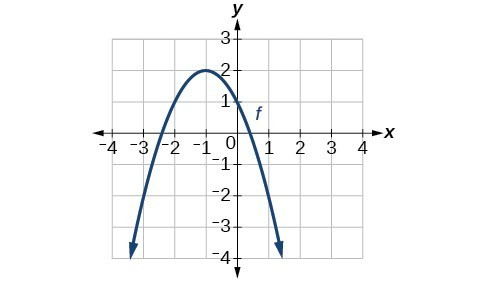44.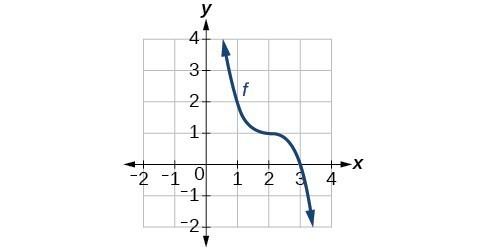45.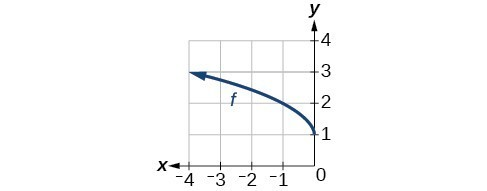46.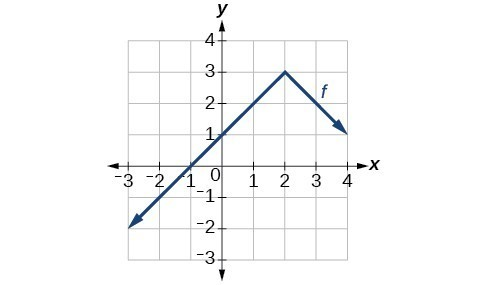For the following exercises, describe how the graph of each function is a transformation of the graph of the original function $f$.

47. $g\left(x\right)=-f\left(x\right)$

48. $g\left(x\right)=f\left(-x\right)$

49. $g\left(x\right)=4f\left(x\right)$

50. $g\left(x\right)=6f\left(x\right)$

51. $g\left(x\right)=f\left(5x\right)$

52. $g\left(x\right)=f\left(2x\right)$

53. $g\left(x\right)=f\left(\frac{1}{3}x\right)$

54. $g\left(x\right)=f\left(\frac{1}{5}x\right)$

55. $g\left(x\right)=3f\left(-x\right)$

56. $g\left(x\right)=-f\left(3x\right)$

For the following exercises, write a formula for the function $g$ that results when the graph of a given toolkit function is transformed as described.

57. The graph of $f\left(x\right)=|x|$ is reflected over the $y$ axis and horizontally compressed by a factor of $\frac{1}{4}$ .

58. The graph of $f\left(x\right)=\sqrt{x}$ is reflected over the $x$ -axis and horizontally stretched by a factor of 2.

59. The graph of $f\left(x\right)=\frac{1}{{x}^{2}}$ is vertically compressed by a factor of $\frac{1}{3}$, then shifted to the left 2 units and down 3 units.

60. The graph of $f\left(x\right)=\frac{1}{x}$ is vertically stretched by a factor of 8, then shifted to the right 4 units and up 2 units.

61. The graph of $f\left(x\right)={x}^{2}$ is vertically compressed by a factor of $\frac{1}{2}$, then shifted to the right 5 units and up 1 unit.

62. The graph of $f\left(x\right)={x}^{2}$ is horizontally stretched by a factor of 3, then shifted to the left 4 units and down 3 units.

For the following exercises, describe how the formula is a transformation of a toolkit function. Then sketch a graph of the transformation.

63. $g\left(x\right)=4{\left(x+1\right)}^{2}-5$

64. $g\left(x\right)=5{\left(x+3\right)}^{2}-2$

65. $h\left(x\right)=-2|x - 4|+3$

66. $k\left(x\right)=-3\sqrt{x}-1$

67. $m\left(x\right)=\frac{1}{2}{x}^{3}$

68. $n\left(x\right)=\frac{1}{3}|x - 2|$

69. $p\left(x\right)={\left(\frac{1}{3}x\right)}^{3}-3$

70. $q\left(x\right)={\left(\frac{1}{4}x\right)}^{3}+1$

71. $a\left(x\right)=\sqrt{-x+4}$

For the following exercises, use the graph below to sketch the given transformations.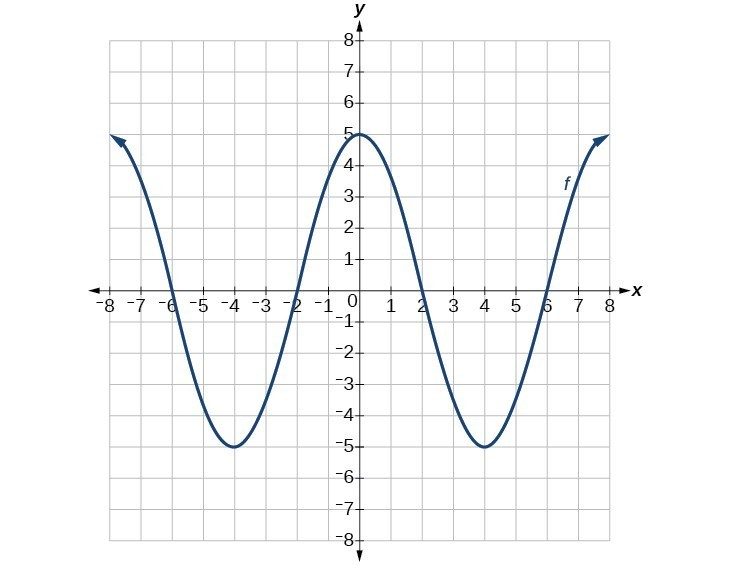72. $g\left(x\right)=f\left(x\right)-2$

73. $g\left(x\right)=-f\left(x\right)$

74. $g\left(x\right)=f\left(x+1\right)$

75. $g\left(x\right)=f\left(x - 2\right)$